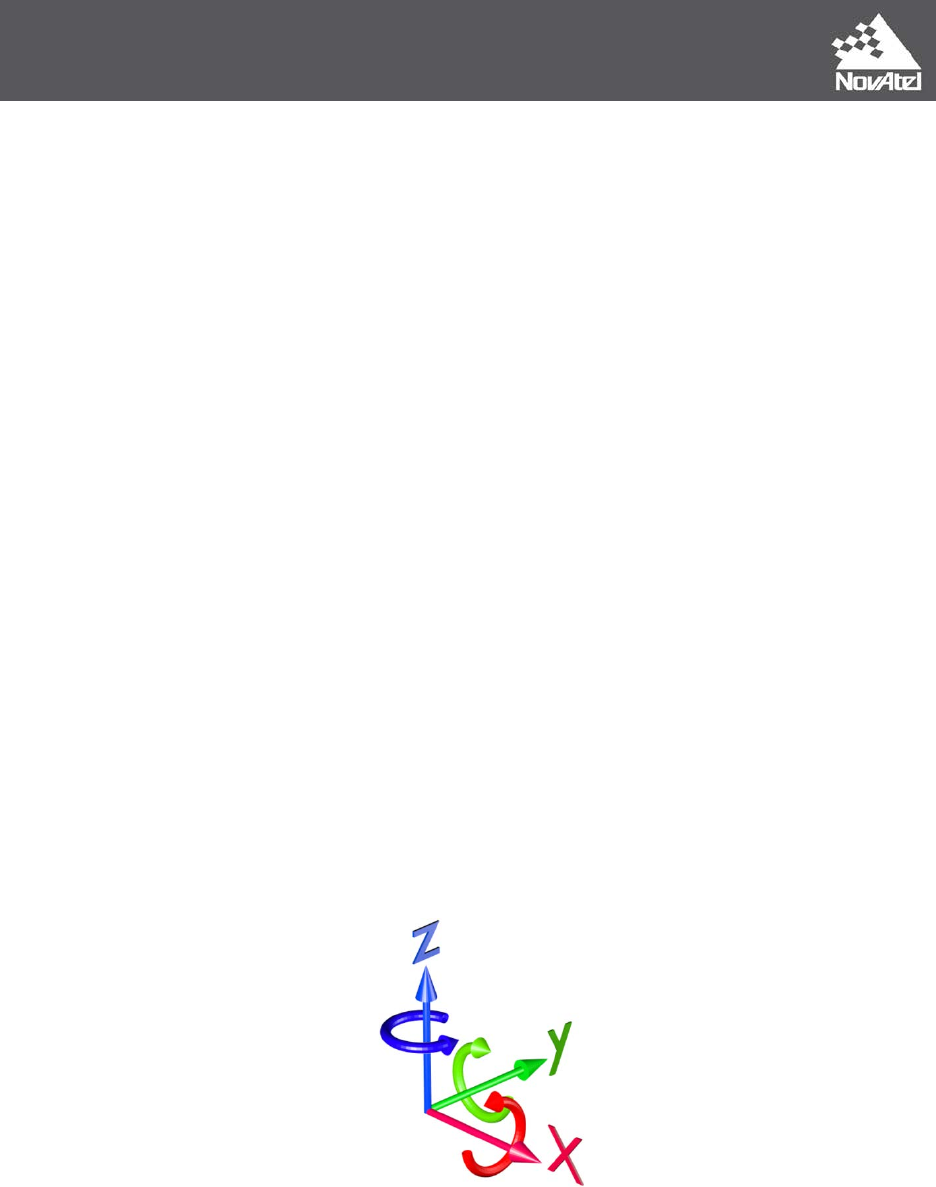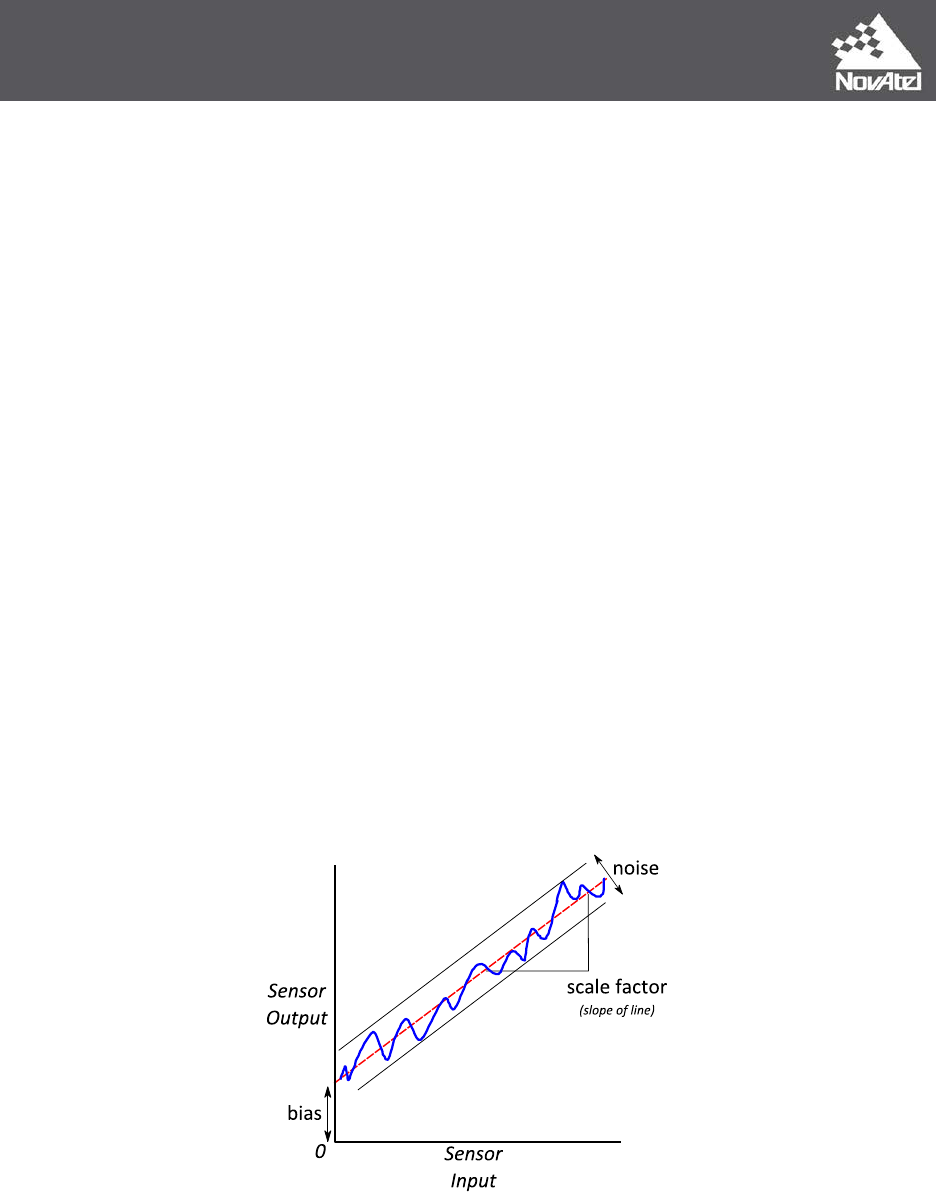Page | 1 February 21, 2014
APN-064 Rev A
IMU Errors and Their Effects
Introduction
An Inertial Navigation System (INS) uses the output from an Inertial Measurement Unit (IMU),
and combines the information on acceleration and rotation with initial information about
position, velocity and attitude. It then delivers a navigation solution with every new
measurement (mechanization).
IMU: Inertial Measurement Unit
Mechanization: Summation of acceleration and attitude rate over time to produce
position, velocity and attitude.
IMU Components
An IMU is typically composed of the following components:
Three accelerometers
Three gyroscopes
Digital signal processing hardware/software
Power conditioning
Communication hardware/software
An enclosure
Three accelerometers are mounted at right angles to each other, so that acceleration can be
measured independently in three axes: X, Y and Z. Three gyroscopes are also at right angles
to each other, so the angular rate can be measured around each of the acceleration axes.
Figure 1: IMU Axes
Signals from the accelerometers and gyroscopes are processed through signal processing at
a very high rate. The measurements are then summed, giving a total acceleration and
rotation for the IMU sample period. For example, in a 200 Hz IMU, the sample period
represents the total motion of the IMU over 5 milliseconds.Page | 2 February 21, 2014
The information about the motion of the IMU is transmitted to the INS. These
measurements are used as input into the INS filter. The end result of combining the IMU
information with GNSS information is position, velocity and attitude. IMU measurements are
integrated with respect to time, as part of the INS filter, so any errors in the measurements
grow over time. To reduce the effect of the measurement errors, they must be understood,
estimated and then corrected.
The Effect of IMU Errors
A GNSS+INS solution combines the measurements from an IMU with satellite positioning
information for a combined solution. The combined solution benefits from the strengths of
each approach to the position problem. The inherent error in IMU measurements, if not
correctly dealt with or estimated as part of an integrated GNSS+INS, can cause significant
problems in the solution.
A bias error, if not removed from the measurement, is integrated twice as part of the
mechanization process. In this case, a constant bias (error) in acceleration becomes a linear
error in velocity and a quadratic error in position. A constant bias in attitude rate (gyro)
becomes a quadratic error in velocity and a cubic error in position.
A converged and well designed INS filter, estimates and removes errors from the IMU
measurements, leading to higher attitude accuracy and longer solution stability during
periods of poor GNSS.
General Error Terms
Repeatability
The ability of the sensor to deliver the same output for the same repeated input, assuming
all other conditions are the same (see Turn-on to Turn-on Bias).
Stability
The ability of the sensor to deliver the same output, over time, for the same constant input.
Drift
The change of the output over time (zero drift is the change over time with no input).Page | 3 February 21, 2014
Measurements When Stationary
Gravity and Earth’s Rotation
Even when an IMU is stationary, it still measures forces. These measurements are the result
of the IMU measuring forces in an inertial frame. The inertial frame is a reference, fixed in
space and time. The Earth moves through the inertial frame and can be seen as experiencing
motion compared to the inertial frame.
Gravity acts in the inertial frame. The strong effect of gravity’s acceleration (~9.8 m/s
2
) can
be measured by the accelerometers (and somewhat on the gyroscopes) and is always
significant when operating near the Earth’s surface.
The rotation of the Earth through the inertial frame is seen in the gyroscope measurements.
Relatively speaking, the effect of the Earth’s rotation is less noticeable and less measurable
with a magnitude of 15 degrees/hr. A perfectly horizontal gyroscope, located at the equator
and pointed north or south, measures the full amount of the Earth’s rotation, but the same
horizontal gyroscope, located at a pole, measures no part of the Earth’s rotation.
To understand how an INS moves through the Earth’s coordinate system (latitude, longitude,
altitude), these forces must be removed from the measurements leaving only the forces due
to motion over the Earth’s surface.
IMU Errors
Not all of these errors are relevant for all IMUs. Some of the error terms are too small to
create a significant difference in the final solution and some of the error terms are never
described by the manufacturer.
A key to having a high performance INS is to understand what the errors are in the system
and developing ways to reduce or remove the errors and error sources.
Figure 2: Common IMU ErrorsPage | 4 February 21, 2014
Input Range 𝒅𝒆𝒈 𝒔
, 𝒈
The input range is the maximum angular rate or acceleration the IMU can meaningfully
measure. Acceleration or rotation outside this range results in bad measurements or no
measurements. It is important to keep this in mind when selecting an IMU, especially in
environments that experience high dynamics or with IMUs which have low input ranges. The
input range can also be described in terms of shock (linear acceleration with respect to time)
or angular acceleration (angular rate with respect to time).
Importantly, strong vibration can lead to a poor solution, as the sensors are already
saturated with signal. The actual signal of vehicle motion (or the body that is the object of
the measurement) is more difficult to separate from the noise caused by the vibration. The
bandwidth of the sensors plays an important part in the ability of the sensors to measure
actual motion. A low bandwidth means high frequency vibration is not properly measured,
so the resulting measurement suffers from aliasing. For this reason, vibration isolation is
recommended in situations where significant vibration is present or with low bandwidth
sensors.
Bias 𝒅𝒆𝒈 𝒉𝒓
, 𝒎 𝒔
𝟐
For a given physical input, the sensor outputs a measurement, offset by the bias. For
example, if the IMU is stationary and level, the vertical axis measures the effect of
gravitational acceleration. Gravity has a nominal acceleration of 9.81 m/s^2, but if the
measurement is biased, the IMU may report 9.75 m/s^2. The difference between the real
value and the output is the bias. In the single axis equation, the bias is denoted as b.
𝑥 = 𝑆𝑓
(
𝑥
)
+ 𝑏
Bias can be broken into two components that describe the behavior of the bias over time;
repeatability and stability.
Bias Repeatability (Turn-on to Turn-on Bias) 𝒅𝒆𝒈 𝒉𝒓
, 𝒎 𝒔
𝟐
For each powerup of the IMU, the initial bias is different. This is due to a number of effects,
including change in the physical properties of the IMU and initial conditions of signal
processing. A very repeatable bias allows for better "tuning" of IMU parameters by the INS
to quickly reach a good estimate of the bias. A high variability in the turn-on to turn-on bias,
leads to a longer and more difficult estimation period (convergence) for every start up.Page | 5 February 21, 2014
Bias Stability (In-run Bias) 𝒅𝒆𝒈 𝒉𝒓
𝒉𝒓
, 𝒎 𝒔
𝟐
𝒉𝒓
While the IMU is powered on, the initial bias changes over time. This change in bias is often
related to temperature, time and/or mechanical stress on the system. In the case of light
based gyroscopes (Fibre Optic Gyro (FOG)/Ring Laser Gyro (RLG)), the optical length
increases or decreases with the change in the physical properties of the IMU. Often, IMUs
are manufactured with temperature compensation, increasing the stability of the
measurements.
An INS filter constantly estimates the bias by making use of external sources of information
(GNSS, DMI, barometer). The estimated bias value is removed from the IMU measurements
before using them in the mechanization.
The process of estimating the bias is more effective when stable. The effect of bias stability
can be observed directly in the outage performance.
Scale Factor 𝒑𝒑𝒎
Scale factor error is the relation between input and output. If the input is 100%, the
expected output is 100%. The actual output is the result of a linear effect, where the output
is proportional to the input but scaled. For example, if the input is 10 m/s
2
, but there is a 2%
scale factor error, the output measurement is 10.2 m/s
2
. This can also be described as a
20000 ppm error. Another way to think about scale factor is the slope of the sensor signal,
see Figure 2: Common IMU Errors. In the following single axis equation, the scale factor is
denoted as S.
𝑥 = 𝑆𝑓
(
𝑥
)
+ 𝑏
Scale Factor Linearity 𝒑𝒑𝒎
Linearity is a further consideration for scale factor. The non-linear part of the scale factor
effect is described by this number. Quite often, IMU manufacturers combine the linear and
non-linear parts of the scale factor into one value.
Scale factor effects are most apparent in times of high acceleration and rotation.
Random Walk (Sensor Noise) 𝒅𝒆𝒈
𝒉𝒓
, 𝒎/𝒔
𝒉𝒓
If a sensor measures a constant signal, a random noise (error) in the measurement is always
present. This is described as a stochastic process and is minimized using statistical
techniques. The integration (mechanization) of the random walk errors in the
measurements, leads to a random walk in the final solution. One area where the random
walk of the gyroscopes plays an important role is in static alignment. The quality of the static
alignment result is directly related to the noise of the sensors.
Random walk has a direct effect on the performance of a GNSS+INS during periods of GNSS
outage, when the sensor noise error causes the solution error to grow unbounded.Page | 6 February 21, 2014
Sensor Non-orthogonality (Misalignment) 𝒎𝒓𝒂𝒅
The three gyroscopes and three accelerometers are mounted orthogonal to each other. The
mountings, however, have errors and so are not perfectly 90 degrees. This leads to a
correlation between sensors. For example, assume one axis is pointed perfectly up and the
IMU is level. The accelerometer on this axis is measuring gravity. If the other two axes were
perfectly orthogonal, they do not measure any of the effect of gravity. If there is a non-
orthogonality, the other axes also measure gravity, leading to a correlation in the
measurements.
The effect of non-orthogonality occurs within sensor sets (between accelerometers or
gyroscopes), between sensor sets or between the sensor sets and the enclosure (package
misalignment). Careful manufacturing, as well as factory calibration, can help minimize this
error source. Continuous estimation and correction during system operation is also an
approach used to minimize this effect.
Package misalignment (between the IMU and the enclosure) can be removed by performing
a boresighting estimation to determine the offset between the IMU measurement frame
and the sensor (objective) frame.
G Dependency (Acceleration Effect) 𝒅𝒆𝒈 𝒉𝒓
, 𝒎 𝒔
𝟐
Some gyroscopes and accelerometers are subject to a change in the bias depending on how
the sensor experiences acceleration. This is most common in Micro Electro Mechanical
Systems (MEMS) gyroscopes, when the mass undergoes acceleration along the sensing axis.
This effect can be modeled and removed from the measurements and is often included in
the IMU signal condition stage before output of the measurements.
Timing Errors (Latency)
The difference between the time the IMU measures motion and the time that external
sources like GNSS measure the same motion, is a very important factor in the quality of the
resulting, combined solution. When the INS+GNSS timing disagree, by large enough factors,
errors become apparent, especially in dynamic situations.
Integrated INS equipment corrects for this difference in measurement time automatically,
and removes this error from the output measurements and final navigation solution.